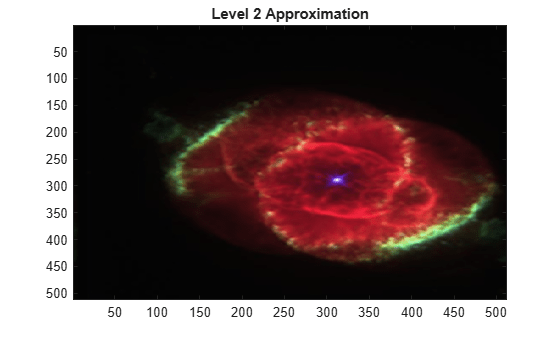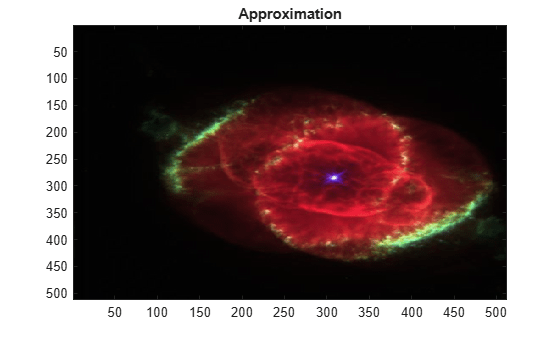# iswt2

Inverse discrete stationary wavelet transform 2-D

## Syntax

```X = iswt2(SWC,'wname') X = iswt2(A,H,V,D,wname) X = iswt2(A(:,:,end),H,V,D,'wname') X = iswt2(A(:,:,1,:),H,V,D,'wname') X = iswt2(SWC,Lo_R,Hi_R) X = iswt2(A,H,V,D,Lo_R,Hi_R) X = iswt2(A(:,:,end),H,V,D,Lo_R,Hi_R) X = iswt2(A(:,:,1,:),H,V,D,'wname') ```

## Description

`iswt2` performs a multilevel 2-D stationary wavelet reconstruction using either an orthogonal or a biorthogonal wavelet. Specify the wavelet using its name (`'wname'`, see `wfilters` for more information) or its reconstruction filters (`Lo_R` and `Hi_R`).

`X = iswt2(SWC,'wname')` or ```X = iswt2(A,H,V,D,wname)``` reconstructs the signal `X`, based on the multilevel stationary wavelet decomposition structure `SWC` or `[A,H,V,D]` (see `swt2`).

If multilevel stationary wavelet decomposition structure `SWC` or `[A,H,V,D]` was generated from a 2-D matrix, the syntax `X = iswt2(A(:,:,end),H,V,D,'wname')` reconstructs the signal `X`.

If the stationary wavelet decomposition structure `SWC` or `[A,H,V,D]` was generated from a single level stationary wavelet decomposition of a 3-D matrix, `X = iswt2(A(:,:,1,:),H,V,D,'wname')` reconstructs the signal `X`.

`X = iswt2(SWC,Lo_R,Hi_R)` or ```X = iswt2(A,H,V,D,Lo_R,Hi_R)``` or `X = iswt2(A(:,:,end),H,V,D,Lo_R,Hi_R)` or `X = iswt2(A(:,:,1,:),H,V,D,'wname')` reconstructs as in the previous syntax, using filters that you specify:

• `Lo_R` is the reconstruction low-pass filter.

• `Hi_R` is the reconstruction high-pass filter.

`Lo_R` and `Hi_R` must be the same length.

### Note

• `iswt2` synthesizes `X` from the coefficient arrays generated by `swt2`. `swt2` uses double-precision arithmetic internally and returns double-precision coefficient matrices. `swt2` warns if there is a loss of precision when converting to double.

• To distinguish a single-level decomposition of a truecolor image from a multilevel decomposition of an indexed image, the approximation and detail coefficient arrays of truecolor images are 4-D arrays. See Distinguish Single-Level Truecolor Image from Multilevel Indexed Image Decompositions. Also see examples Stationary Wavelet Transform of an Image and Inverse Stationary Wavelet Transform of an Image.

If an `K`-level decomposition is performed, the dimensions of the `A`, `H`, `V`, and `D` coefficient arrays are `m`-by-`n`-by-3-by-`K`.

If a single-level decomposition is performed, the dimensions of the `A`, `H`, `V`, and `D` coefficient arrays are `m`-by-`n`-by-1-by-3. Since MATLAB®removes singleton last dimensions by default, the third dimension of the coefficient arrays is singleton.

## Examples

collapse all

Demonstrate perfect reconstruction using `swt2` and `iswt2` with an orthogonal wavelet.

```load woman [Lo_D,Hi_D,Lo_R,Hi_R] = wfilters('db6'); [ca,chd,cvd,cdd] = swt2(X,3,Lo_D,Hi_D); recon = iswt2(ca,chd,cvd,cdd,Lo_R,Hi_R); norm(X-recon)```
```ans = 1.0126e-08 ```

In this example you obtain single-level and multilevel stationary wavelet decompositions of a truecolor image. You view results of each decomposition.

Load in a truecolor image. The image is a 3-D array of type `uint8`. Since `swt2` requires the first and second dimensions both be divisible by a power of 2, extract a portion of the image and view it.

```imdata = imread('ngc6543a.jpg'); x = imdata(1:512,1:512,:); imagesc(x)```Obtain the 4-level stationary wavelet decomposition of the image using the `db4` wavelet. Return the approximation coefficients and the horizontal, vertical, and detail coefficients as separate arrays. Note the dimensions of the output arrays.

```[a,h,v,d] = swt2(x,4,'db4'); size(a)```
```ans = 1×4 512 512 3 4 ```
`size(h)`
```ans = 1×4 512 512 3 4 ```
`size(v)`
```ans = 1×4 512 512 3 4 ```
`size(d)`
```ans = 1×4 512 512 3 4 ```

The output arrays are all of type `double`. View the level 2 approximation coefficients. Since the approximation coefficients are of type `double`, cast them as `uint8`, which is the datatype of the image.

```figure imagesc(uint8(a(:,:,:,2))) title('Level 2 Approximation Coefficients')```Reconstruct the image by performing the inverse transform. Compute the difference between the original image and reconstruction. Since the reconstruction is of type `double`, cast the reconstruction as type `uint8` before computing the difference.

```rec = iswt2(a,h,v,d,'db4'); maxdiff = max(abs(uint8(rec(:))-x(:))); disp(['maximum difference = ' num2str(maxdiff)])```
```maximum difference = 0 ```

Obtain the single-level stationary wavelet decomposition of the image using the `db4` wavelet. Return the approximation coefficients and horizontal, vertical, and detail coefficients in separate arrays. Note the dimensions of the output arrays.

```[a,h,v,d] = swt2(x,1,'db4'); size(a)```
```ans = 1×4 512 512 1 3 ```
`size(h)`
```ans = 1×4 512 512 1 3 ```
`size(v)`
```ans = 1×4 512 512 1 3 ```
`size(d)`
```ans = 1×4 512 512 1 3 ```

View the approximation coefficients. To prevent an error when using `imagesc`, squeeze the approximation coefficients array to remove the singleton dimension.

```asqueeze = squeeze(a); size(asqueeze)```
```ans = 1×3 512 512 3 ```
```figure imagesc(uint8(asqueeze)) title('Approximation Coefficients')```Reconstruct the image by performing the inverse transform. Compute the difference between the original image and reconstruction. Since the reconstruction is of type `double`, cast the reconstruction as type `uint8` before computing the difference.

```rec = iswt2(a,h,v,d,'db4'); maxdiff = max(abs(uint8(rec(:))-x(:))); disp(['maximum difference = ' num2str(maxdiff)])```
```maximum difference = 0 ```

This example shows how to reconstruct a truecolor image from a single-level stationary wavelet decomposition using 3-D approximation and detail coefficient arrays.

Load in a truecolor image. The image is a 3-D array of type `uint8`. Since `swt2` requires the first and second dimensions both be divisible by a power of 2, extract a portion of the image and view it.

```imdata = imread('ngc6543a.jpg'); x = imdata(1:512,1:512,:); imagesc(x)```Obtain the single-level stationary wavelet decomposition of the image using the `db4` wavelet. Return the approximation coefficients and horizontal, vertical, and detail coefficients in separate arrays. Note the dimensions of the output arrays.

```[a,h,v,d] = swt2(x,1,'db4'); size(a)```
```ans = 1×4 512 512 1 3 ```
`size(h)`
```ans = 1×4 512 512 1 3 ```
`size(v)`
```ans = 1×4 512 512 1 3 ```
`size(d)`
```ans = 1×4 512 512 1 3 ```

Squeeze the coefficient arrays to remove their singleton dimensions. Note the dimensions of the squeezed arrays.

```asq = squeeze(a); hsq = squeeze(h); vsq = squeeze(v); dsq = squeeze(d); size(asq)```
```ans = 1×3 512 512 3 ```
`size(hsq)`
```ans = 1×3 512 512 3 ```
`size(vsq)`
```ans = 1×3 512 512 3 ```
`size(dsq)`
```ans = 1×3 512 512 3 ```

So that `iswt2` can properly reconstruct the trueimage from the new coefficient arrays, insert a singleton dimension by reshaping the squeezed arrays. Reconstruct the image with the reshaped coefficient arrays.

```a2 = reshape(asq,[512,512,1,3]); h2 = reshape(hsq,[512,512,1,3]); v2 = reshape(vsq,[512,512,1,3]); d2 = reshape(dsq,[512,512,1,3]); rec = iswt2(a2,h2,v2,d2,'db4');```

Compute the difference between the original image and reconstruction. Since the reconstruction is of type `double`, cast the reconstruction as type `uint8` before computing the difference.

```maxdiff = max(abs(uint8(rec(:))-x(:))); disp(['maximum difference = ' num2str(maxdiff)])```
```maximum difference = 0 ```

## Tips

If SWC or (cA,cH,cV,cD) are obtained from an indexed image analysis or a truecolor image analysis, then X is an `m`-by-`n` matrix or an `m`-by-`n`-by-3 array, respectively.

For more information on image formats, see the `image` and `imfinfo` reference pages.

## Compatibility Considerations

expand all

Behavior changed in R2017b

## References

Nason, G.P.; B.W. Silverman (1995), “The stationary wavelet transform and some statistical applications,” Lecture Notes in Statistics, 103, pp. 281–299.

Coifman, R.R.; Donoho D.L. (1995), “Translation invariant de-noising,” Lecture Notes in Statistics, 103, pp. 125–150.

Pesquet, J.C.; H. Krim, H. Carfatan (1996), “Time-invariant orthonormal wavelet representations,” IEEE Trans. Sign. Proc., vol. 44, 8, pp. 1964–1970.